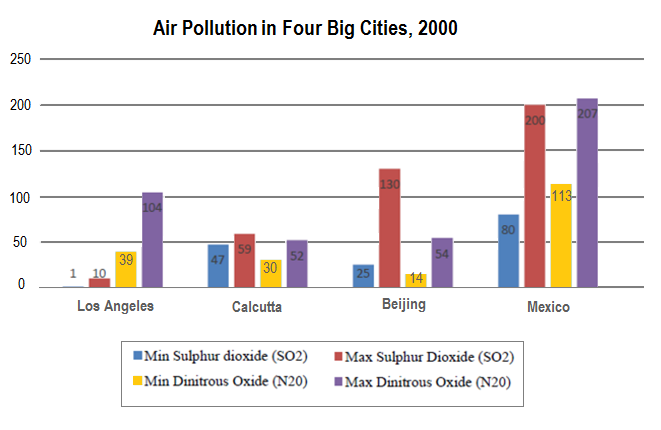## Graph Writing # 191 - Average daily minimum and maximum levels of two air pollutants

### The chart below shows the average daily minimum and maximum levels of two air pollutants in four big cities in 2000.

Summarise the information by selecting and reporting the main features and make comparisons where relevant.

» You should write at least 150 words.The column chart compares the lowest and highest levels of Sulphur Dioxide (SO2) and Dinitrous Oxide (NO2), two air pollutants, in four cities, namely Los Angeles, Calcutta, Beijing and Mexico in 2000.

Overall, the average presence of these two air polluting ingredients was much higher in Mexico than the other three cities. The higher presence of Sulphur Dioxide and Dinitrous Oxide in Mexico reveal that air pollution in this city is worse than the three other cities.

As is presented in the graph, the level of Sulphur Dioxide in the air of Los Angeles ranges from 1 to 10 which is remarkably lower than that of other cities. The presence of this air pollutant varies from 80 to 200 in Mexico which is the highest. The minimum level of SO2 is 47 in Calcutta and 25 in Beijing but the maximum range goes as high as 130 in Beijing which is more than double than that of Calcutta city.

On the other hand, the presence of the second air polluting material - Dinitrous oxide, on an average, could be found more in the Mexico City’s air than any other city provided in the illustration. The minimum level of N2O in Mexico was 113 which was almost ten times higher than that of Beijing and approximately four times higher than that of Calcutta and Los Angeles. The upper level of N2O in Los Angeles was higher than the presence of N2O in Calcutta and Beijing.Nguyen Thuong Vu
The bar chart illustrates the highest and lowest levels of Dinitrous Oxide (N2O) and Sulphur Dioxide (SO2) in Los Angeles, Calcutta, Beijing and Mexico in the year 2000.

Overall, the statistics ranged from as low as 1 to the high of 207. Additionally, it can be seen that the levels of pollutants in Mexico were higher than in the remaining cities.

In detail, the lowest level of SO2 in Mexico was 80, which was more than three times that of Beijing. This figure was 47 in Calcutta and 1 in Los Angeles - almost unnoticeable. At the same time, the range of SO2 levels stood between 10 (Los Angeles) to 200 (Mexico).

Regarding the pollutant N2O, the chart shows that Calcutta had the smallest difference between the lowest and highest level, which were 30 and 52, respectively. Maximum N2O in Beijing represented 54 while this statistic in Los Angeles was almost twice as much, 104.

It is worth noticing that the minimum level of Dinitrous oxide in Mexico even exceeded the maximum figures of this pollutant in the three remaining cities. According to the diagram, the N2O level in this city ranged from 113 to 207 in 2000.

Gurnoor
The provided column graph illustrates the minimum and maximum levels of sulphur dioxide and di-nitrous oxide in four big cities, namely - Los Angeles, Calcutta, Beijing and Mexico, in the year 2000.

At a glance, Mexico showed the high average daily minimum and maximum levels of the two air pollutants.

As it can be observed from the diagram, Los Angeles had a very negligible amount of SO2, and maximum Sulphur dioxide amounts to only 10, whereas minimum levels of Sulphur dioxide in the other three cities were high in comparison - 25 in Beijing, 47 in Calcutta, and 80 in Mexico. Their upper limits were 59, 130, 200, respectively. And the lower limit with regard to the presence of di-nitrous oxide or N2O was the least in the capital city of China (Beijing) with just 14 units and the second position was occupied by Calcutta (30). Then came Los Angeles (39), Mexico (113), sequentially. Maximum levels of di-nitrous oxide were almost double their minimum level in Mexico and Calcutta, about four times in Beijing and just around trebled in Los Angeles.

Bianca
The column chart demonstrates the lowest and the highest levels of two air polluting substances, Sulphur Dioxide (SO2) and Dinitrous Oxide (N2O), in four large cities - Los Angeles, Calcutta, Beijing, and Mexico, during the year 2000.

A glance at the graph reveals that the presence of these two air pollutants was higher in Mexico than the other cities in 2000.

As it can be observed from the graph, the daily level of Sulphur Dioxide in Mexico City varied between 80 and 200 which was significantly higher than that of other cities. While in Beijing the minimum level of this substance was 25 and the maximum 130, in Calcutta this level ranged from 47 to 59 and Los Angeles had the lowest level of SO2 which varied from 1 to 10 only.

Despite it, Los Angeles presented a higher level of N2O than Calcutta and Beijing with 39 as the minimum and 104 as the maximum of Dinitrous Oxide in a day. In Calcutta, it varied between 30 and 52 and, the city of Beijing presented the lowest level that daily ranged from 14 to 54. On the other hand, the average presence of NO2 in Mexican air was the highest of all, between 113 to 207 a day. Overall, it is clear from the chart that Mexico had the highest level of both polluting substances in 200; therefore the air in this city could be considered the worse of them in the period.